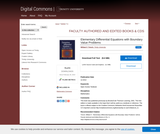# 2 Results

View
Selected filters:
• Trinity UniversityUnrestricted Use
CC BY
Rating

Elementary Differential Equations with Boundary Value Problems is written for students in science, engineering, and mathematics who have completed calculus through partial differentiation. If your syllabus includes Chapter 10 (Linear Systems of Differential Equations), your students should have some preparation in linear algebra. In writing this book I have been guided by the these principles:  An elementary text should be written so the student can read it with comprehension without too much pain. I have tried to put myself in the students place, and have chosen to err on the side of too much detail rather than not enough.  An elementary text cant be better than its exercises. This text includes 2041 numbered exercises, many with several parts. They range in difficulty from routine to very challenging.  An elementary text should be written in an informal but mathematically accurate way, illustrated by appropriate graphics. I have tried to formulate mathematical concepts succinctly in language that students can understand. I have minimized the number of explicitly stated theorems and defonitions, preferring to deal with concepts in a more conversational way, copiously illustrated by 299 completely worked out examples. Where appropriate, concepts and results are depicted in 188 figures

Subject:
Engineering
Mathematics
Material Type:
Textbook
Provider:
Trinity University
Author:
William F. Trench
10/28/2014Conditional Remix & Share Permitted
CC BY-NC-SA
Rating

This is a text for a two-term course in introductory real analysis for junior or senior mathematics majors and science students with a serious interest in mathematics. Prospective educators or mathematically gifted high school students can also benefit from the mathematical maturity that can be gained from an introductory real analysis course.

The book is designed to fill the gaps left in the development of calculus as it is usually presented in an elementary course, and to provide the background required for insight into more advanced courses in pure and applied mathematics. The standard elementary calculus sequence is the only specific prerequisite for Chapters 1–5, which deal with real-valued functions. (However, other analysis oriented courses, such as elementary differential equation, also provide useful preparatory experience.) Chapters 6 and 7 require a working knowledge of determinants, matrices and linear transformations, typically available from a first course in linear algebra. Chapter 8 is accessible after completion of Chapters 1–5.

Subject:
Mathematics
Calculus
Functions
Material Type:
Textbook
Provider:
Trinity University
Provider Set:
Digital Commons @Trinity University
Author:
William Trench